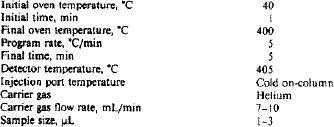ASTM D5480 Standard Test Method for Engine Oil Volatility by Gas chromatography
8. Procedure
8.1 Prepare the internal standard mixture by adding 10 mL each of decane, undecane, and dodecane to a stoppered flask, and mix well.

8.2 Prepare the tetracosane solution by adding 0.2 g tetracosane to a 100-mL volumetric flask and diluting to the mark with carbon disulfide (Solution A).

8.3 Weigh 2.0 g engine oil and 0.1 g of internal standard mixture (each to the nearest 0.1 mg) into a 25-mL volumetric flask and dilute to the mark with carbon disulfide (Solution B).

8.4 Using a pipet, add 1 mL of Solution A and l mL of Solution B to a 10-mL volumetric flask and dilute to the mark with carbon disulfide (Solution C).

8.5 Inject an aliquot of solution c into the gas chromatograph using the instrument parameters given in 8.6. An injection technique should be chosen which does not cause distortion of the peak shapes associated with the performance standard prepared in 7.2. They can vary depending upon the instrument and column utilized. The final column temperature should not exceed the recommendations of the manufacturer, but should be high enough to ensure elution of the oil. A typical chromatogram is shown in Fig.2.

8.6 Recommended instrument Operating parameters:9. Calculation
9.1 Tabulate the retention times observed for decane, undecane, dodecane, and tetracosane versus their respective boiling points, and calculate the retention time equivalent to 371°C (700°F) using linear regression and interpolation.
9.1.1 Although descriptions of how to apply linear regression can be found in many mathematical textbooks, scientific hand calculators are readily available that will perform the operation.

9.1.2 Refer to Table 1 for boiling points of n-paraffins.

9.2 Determine the mass percentage of engine oil volatilized to 371°C as follows:
A = 100 BC/DE
where:
A = engine oil volatilized to 371°C, mass, %,
B = area of the engine oil up to 371°C,
c = mass of internal standard added, g,
D = total area of the three internal standard peaks,
E = mass of total engine oil, g, and
100 = factor to convert g/g to mass, %.